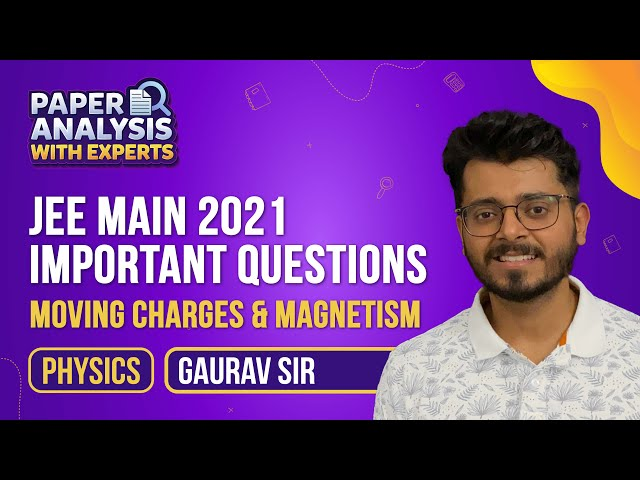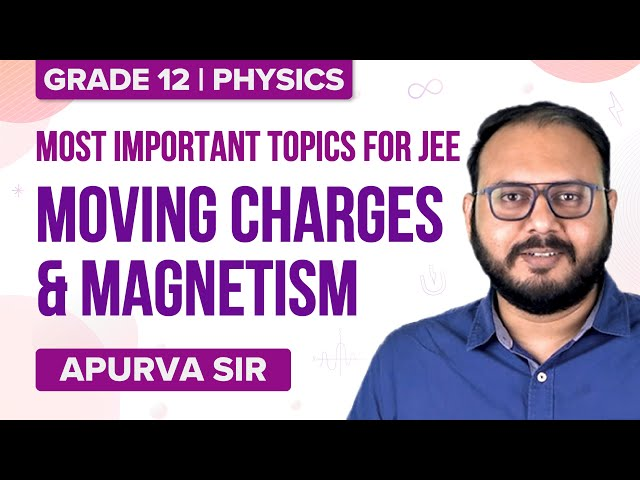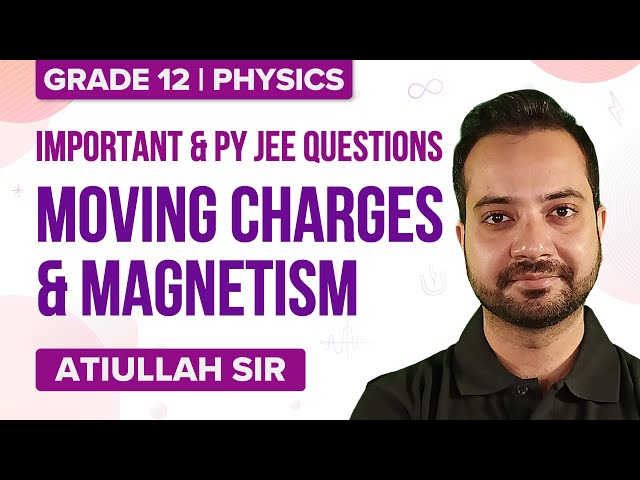# Class 12 Physics Chapter 4 Moving Charges and Magnetism MCQs

Class 12 Physics Chapter 4 Moving Charges and Magnetism MCQs are provided here with answers. These questions are designed as per the latest CBSE syllabus and NCERT curriculum. Solving these chapter-wise MCQs will help students to score good marks in the final exam. Moving Charges and Magnetism Class 12 Physics MCQs are prepared to understand the concept better. It allows students to test their knowledge and answering skills in the given time frame.Download Chapter 4 Moving Charges and Magnetism MCQs PDF by clicking on the button below.

## MCQs on Class 12 Chapter 4 Moving Charges and Magnetism

Check the multiple-choice questions for the 12th Class Physics Moving Charges and Magnetism Chapter. Each MCQ will have four options here, out of which only one is correct. Students have to pick the correct option and check the answer provided here.

1. The magnetic moment of a current I carrying a circular coil of radius r and number of turns N varies as

1. $$\begin{array}{l}r^{4}\end{array}$$
2. $$\begin{array}{l}r^{2}\end{array}$$
3. $$\begin{array}{l}\frac{1}{r^{4}}\end{array}$$
4. r

$$\begin{array}{l}r^{2}\end{array}$$

Explanation: The magnetic moment of a current I carrying a circular coil of radius r and number of turns N varies as

$$\begin{array}{l}r^{2}\end{array}$$
.

2. Magnetic field at the centre of a circular current-carrying conductor/coil is given by

1. $$\begin{array}{l}B=\frac{\mu_{0} I}{2r}\end{array}$$
2. $$\begin{array}{l}B=\frac{\mu_{0}+ I}{2+r}\end{array}$$
3. $$\begin{array}{l}B=\frac{I}{2r}\end{array}$$
4. $$\begin{array}{l}B=\frac{\mu_{0}r I}{2}\end{array}$$

$$\begin{array}{l}B=\frac{\mu_{0} I}{2r}\end{array}$$

Explanation: Magnetic field at the centre of a circular current-carrying conductor/coil is given by

$$\begin{array}{l}B=\frac{\mu_{0} I}{2r}\end{array}$$
.

3. SI unit of the magnetic field is ————-

1. Dyne
2. Ohm
3. Tesla
4. Volt

Explanation: The SI unit of the magnetic field is Tesla.

4. When the charged particles move in a combined magnetic and electric field, then the force acting is known as ……………………

1. Centripetal force
2. Centrifugal force
3. Lorentz force
4. Orbital force

Explanation: When the charged particles move in a combined magnetic and electric field, then the force acting is known as Lorentz force.

5. Magnetic field at any point inside the straight solenoid is given as———-

1. B = μ0+nI
2. B = μ0+n+I
3. B = μ0/nI
4. B = μ0nI

Explanation: Magnetic field at any point inside the straight solenoid is given as B = μ0nI.

6. Cyclotron is a device used to ———————-

1. Slow down charged particles
2. Accelerate the positively charged particles
3. Stop the charged particles
4. None of the options

Answer: (b) Accelerate the positively charged particles

Explanation: Cyclotron is a device used to accelerate positively charged particles.

7. 1 Gauss =

1. $$\begin{array}{l}10^{4}\end{array}$$
Tesla
2. $$\begin{array}{l}10^{-4}\end{array}$$
Tesla
3. $$\begin{array}{l}10^{2}\end{array}$$
Tesla
4. $$\begin{array}{l}10^{-2}\end{array}$$
Tesla

$$\begin{array}{l}10^{-4}\end{array}$$
Tesla

Explanation: 1 Gauss =

$$\begin{array}{l}10^{-4}\end{array}$$
Tesla.

8. State true or false: Cyclotron is a device used to accelerate uncharged particles like neutrons.

1. True
2. (b) False

Explanation: A cyclotron is a device used to accelerate positively charged particles.

9. Lorentz force is given by the formula

1. F= q(v + B + E)
2. F= q(v – B – E)
3. F= q(v * B * E)
4. F= q(v * B + E)

Answer: (d) F= q(v * B + E)

Explanation: Lorentz force is given by the formula F= q(v * B + E)

10. The concept of displacement current was introduced by ———–

1. Newton
2. Ampere
3. Maxwell
4. Fleming